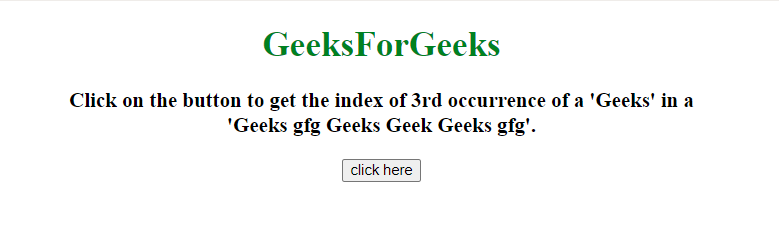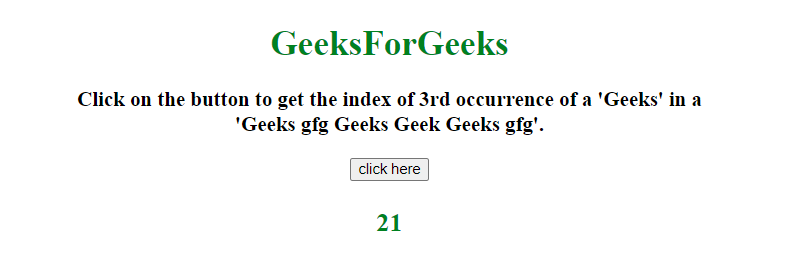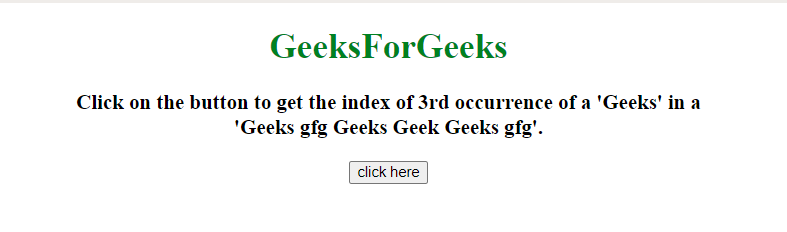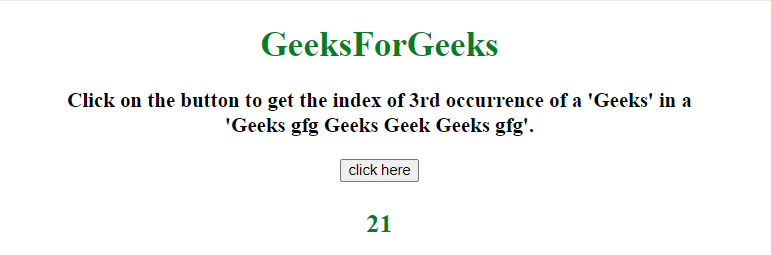# How to get nth occurrence of a string in JavaScript ?

The task is to get the nth occurrence of a substring in a string with the help of JavaScript.

Approach 1:

• First, split the string into sub-strings using split() method by passing index also.
• Again join the substrings on passed substring using join() method.
• Returns the index of nth occurrence of string.

Example:In this example, split() and join() method are used to get the index of substring.

 `  ` `<``html``>  ` ` `  `<``head``>  ` `    ``<``title``>  ` `        ``How to get nth occurrence of ` `        ``a string in JavaScript ? ` `    `` ` `  ` ` `  `<``body` `style` `= ``"text-align:center;"``>  ` `     `  `    ``<``h1` `style` `= ``"color:green;"` `>  ` `        ``GeeksForGeeks ` `    `` ` `     `  `    ``<``p` `id` `= ``"GFG_UP"` `style` `=  ` `        ``"font-size: 19px; font-weight: bold;"``> ` `    `` ` `     `  `    ``<``button` `onclick` `= ``"GFG_Fun()"``> ` `        ``click here ` `    `` ` `     `  `    ``<``p` `id` `= ``"GFG_DOWN"` `style` `=  ` `        ``"color: green; font-size: 24px; font-weight: bold;"``> ` `    `` ` `     `  `    ``<``script``> ` `        ``var el_up = document.getElementById("GFG_UP"); ` `        ``var el_down = document.getElementById("GFG_DOWN"); ` `        ``var string = "Geeks gfg Geeks Geek Geeks gfg"; ` `        ``var searchString = 'Geeks'; ` `        ``var occurrence = 3; ` `         `  `        ``el_up.innerHTML = "Click on the button to get the index of " ` `                ``+ occurrence + "rd occurrence of a '" + searchString ` `                ``+ "' in a <``br``>'" + string + "'."; ` `         `  `        ``function getPos(str, subStr, i) { ` `            ``return str.split(subStr, i).join(subStr).length; ` `        ``} ` `        ``function GFG_Fun() { ` `            ``el_down.innerHTML ` `                    ``= getPos(string, searchString, occurrence); ` `        ``} ` `    ``  ` ` ` ` `  ` `

Output:

• Before clicking on the button:• After clicking on the button:Approach 2: Go through each substring one by one and return the index of the last substring. This approach uses indexOf() method to return the index of nth occurrence of the string.

Example:

 `  ` `<``html``>  ` ` `  `<``head``>  ` `    ``<``title``>  ` `        ``How to get nth occurrence of ` `        ``a string in JavaScript ? ` `    `` ` `  ` ` `  `<``body` `style` `= ``"text-align:center;"` `id` `= ``"body"``>  ` `     `  `    ``<``h1` `style` `= ``"color:green;"` `>  ` `        ``GeeksForGeeks ` `    `` ` `     `  `    ``<``p` `id` `= ``"GFG_UP"` `style` `=  ` `        ``"font-size: 19px; font-weight: bold;"``> ` `    `` ` `     `  `    ``<``button` `onclick` `= ``"GFG_Fun()"``> ` `        ``click here ` `    `` ` `     `  `    ``<``p` `id` `= ``"GFG_DOWN"` `style` `= ` `        ``"color: green; font-size: 24px; font-weight: bold;"``> ` `    `` ` `     `  `    ``<``script``> ` `        ``var el_up = document.getElementById("GFG_UP"); ` `        ``var el_down = document.getElementById("GFG_DOWN"); ` `        ``var string = "Geeks gfg Geeks Geek Geeks gfg"; ` `        ``var searchString = 'Geeks'; ` `        ``var occurrence = 3; ` `         `  `        ``el_up.innerHTML = "Click on the button to get the index of " ` `                ``+ occurrence + "rd occurrence of a '" + searchString ` `                ``+ "' in a <``br``>'" + string + "'."; ` `         `  `        ``function getIndex(str, substr, ind) { ` `            ``var Len = str.length, i = -1; ` `            ``while(ind-- && i++ < ``Len``) { ` `                ``i` `= ``str``.indexOf(substr, i); ` `                ``if (i < 0) break; ` `            ``} ` `            ``return i; ` `        ``} ` `        ``function GFG_Fun() { ` `            ``el_down.innerHTML`  `                    ``= ``getIndex``(string, searchString, occurrence); ` `        ``} ` `    ``  ` ` ` ` `  ` `

Output:

• Before clicking on the button:• After clicking on the button:My Personal Notes arrow_drop_upCheck out this Author's contributed articles.

If you like GeeksforGeeks and would like to contribute, you can also write an article using contribute.geeksforgeeks.org or mail your article to contribute@geeksforgeeks.org. See your article appearing on the GeeksforGeeks main page and help other Geeks.

Please Improve this article if you find anything incorrect by clicking on the "Improve Article" button below.

Improved By : nidhi_biet, Akanksha_Rai